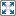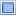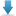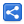# Java - Yielding Thread Execution

[Last Updated: Feb 7, 2017]

The static method `Thread#yield()` causes the current thread to yield its current use of a processor.

In other words the current thread may stop executing momentarily to give chance to other competing threads having the same priorities.

Let's see that with an example.

```public class ThreadYieldExample {
public static void main (String[] args) {

}

private static class Task implements Runnable {
private int c;

@Override
public void run () {
.getName();

for (int i = 0; i < 1000; i++) {
c++;
}

}
}
}/```

In above example we are invoking two threads simultaneously with same priories.

The output is not predictable i.e. we cannot guess which thread is going to take more CPU time and hence faster than the other.

Running above code multiple times:

```Thread-0 started.
```

OR

```Thread-1 started.
```

Let's modify our above code to use yield method for one of the thread:

```public class ThreadYieldExample {
public static void main (String[] args) {

}

private static class Task implements Runnable {
private final boolean shouldYield;
private int c;

this.shouldYield = shouldYield;
}
@Override
public void run () {
.getName();

for (int i = 0; i < 1000; i++) {
c++;
if(shouldYield){
}
}

}
}
}```

In above example we are yielding the first thread in favor of other. As a result the first thread spends less CPU time and it becomes slower than the other, hence 'Thread-0 ended.' printed at the end all the time

Output

```Thread-0 started.
```

Just like thread priorities, yield method behavior varies from OS to OS. We shouldn't make coding decisions based on this method. Also this is only a hint, the thread scheduler (on OS level) is free to ignore this hint.

## Example Project

Dependencies and Technologies Used:

• JDK 1.8
• Maven 3.0.4

 Java Thread Yield ExampleSelect AllDownloadShare